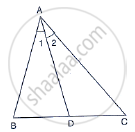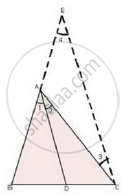Share
Notifications

View all notifications
Books Shortlist
Your shortlist is empty

# Solution for In Triangle Abc , If ∠1 = ∠2, Prove that "Ab"/"Ac"="Bd"/"Dc" - CBSE Class 10 - Mathematics

Login
Create free account

Forgot password?

#### Question

In ΔABC , if ∠1 = ∠2, prove that "AB"/"AC"="BD"/"DC"#### SolutionGiven: A ΔABC in which ∠1 = ∠2

To prove: "AB"/"AC"="BD"/"DC"

Construction: Draw CE || DA to meet BA produced in E.

Proof: since, CE || DA and AC cuts them.

∴ ∠2 − ∠3 …. (i) [Alternate angles]

And, ∠1 − ∠4 ….(ii) [Corresponding angles]

But, ∠1 − ∠2 [Given]

From (i) and (ii), we get

∠3 − ∠4

Thus, in ΔACE, we have

∠3 = ∠4

⇒ AE = AC … (iii) [Sides opposite to equal angles are equal]

Now, In ΔBCE, we have

DA || CE

rArr"BD"/"DC"="BA"/"AE"    [Using basic proportionality theorem]

rArr"BD"/"DC"="AB"/"AC"     [∵ BA – AB and AE – AC from (iii)]

Hence, "AB"/"AC"="BD"/"DC"

Is there an error in this question or solution?

#### Video TutorialsVIEW ALL 

Solution In Triangle Abc , If ∠1 = ∠2, Prove that "Ab"/"Ac"="Bd"/"Dc" Concept: Angle Bisector.
S